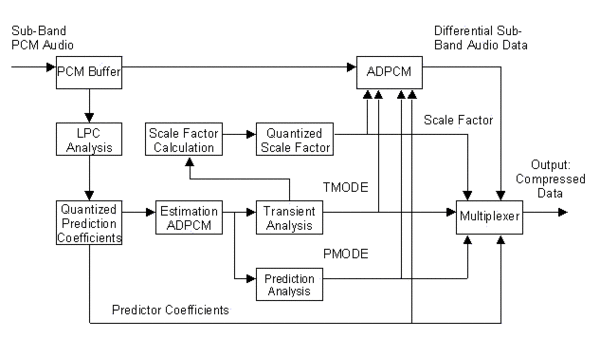Adaptive differential coding (ADPCM) is performed in each sub-band. The result of the coding is a de-correlated sub-band signal by transforming it into a time domain difference signal.The encoding process is based on a subtraction of a linear predictive value (LPC) from the input PCM signal. The resulting difference signal is then quantized and transmitted to the decoder. At the decoder the predicted signal, which was removed at the encoder, can be exactly regenerated and added back to the residual signal, thereby recreating the original input signal.

With a high correlation in the signal, a good prediction of the value of each sample can be made. On subtraction of the prediction from the input signal, small errors are generated which are significantly smaller than the input signal, and hence can be more efficiently re-quantized than the original PCM signal. Otherwise a poor prediction creates a big difference signal that cannot be quantized more efficiently than the original signal.

The ADPCM process is only effective for correlated signals. Thus, the process can be independently switched on or off in each of the 32 sub bands. Therefore an estimation of the coding gain is performed. If this gain is too low, the ADPCM process is switched off.

The audio data passes two ADPCM coding loops. The first one is an estimation loop to create all necessary side information that accompanies the coded audio data to be transmitted to the decoder. The second stage then provides the ?real? ADPCM coding process. In a final step all information is packed by a multiplexer to a defined DTS stream.

A fourth-order forward-adaptive linear predictive coding (LPC analysis) is used to generate the prediction coefficients by analyzing the current audio samples.

In order to reconstruct the predicted signal at the decoder these predictor coefficients must be transmitted to the decoder. So the coefficients are additionally packed to the transmission stream by the multiplexer. The coefficients are transmitted only for those sub-bands, in which the ADPCM process is active. If the ADPCM process is disabled, the coefficients are not transmitted.